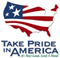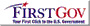Open-File Report 2006–1058

U.S. GEOLOGICAL SURVEY
Open-File Report 2006–1058

## Conversion Factors and Datums

### Conversion Factors

Multiply By To obtain
acre-foot (acre-ft) 0.001233 cubic hectometer (hm3)
cubic foot per second (ft3/s) 0.02832 cubic meter per second (m3/s)
foot (ft) 0.3048 meter (m)
gallon per day (gal/d) 0.003785 cubic meter per day (m3/d)
gallon per minute (gal/min) 0.06309 liter per second (L/s)
inch (in.) 2.54 centimeter (cm)
inch (in.) 25.4 millimeter (mm)
mile (mi) 1.609 kilometer (km)
square mile (mi2) 2.590 square kilometer (km2)

Temperature in degrees Celsius (°C) may be converted to degrees Fahrenheit (°F) as follows:

°F=(1.8×°C)+32.

Specific conductance is given in microsiemens per centimeter at 25 degrees Celsius (µS/cm at 25°C).

Concentrations of chemical constituents in water are given either in milligrams per liter (mg/L) or micrograms per liter (µg/L).

### Datums

Vertical coordinate information is referenced to the National Geodetic Vertical Datum of 1929 (NGVD 29).

Horizontal coordinate information is referenced to the North American Datum of 1927 (NAD 27).

Elevation, as used in this report, refers to distance above the vertical datum.U.S. Department of the Interior | U.S. Geological Survey